Function Repository Resource:

# ChiSquareRandomnessTest

Test a sequence of zeroes and ones (or a set of random reals between 0 and 1) for equidistribution and return a p-value

Contributed by: Emmy/Noah Blumenthal
noahb320@gmail.com
emmyb320@bu.edu
 ResourceFunction["ChiSquareRandomnessTest"][sequence] tests a sequence of 0s and 1s, or random reals between 0 and 1, and returns a p-value. ResourceFunction["ChiSquareRandomnessTest"][sequence,"property"] tests a sequence and returns a a property of the test.

## Details and Options

Properties include:
 "TestStatistic" returns the chi square test statistic "PValue" returns the p-value associated with the test
The bin size for testing random reals is 1/n where n is the sequence length.
The chi-square randomness test works best for larger sequences of numbers.
The chi-square randomness test only tests for equidistribution.

## Examples

### Basic Examples (3)

Generate a sequence of random integers and test it for equidistribution:

 In:=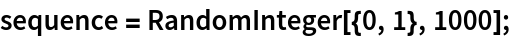Visualize the sequence:

 In:=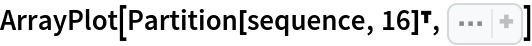Out=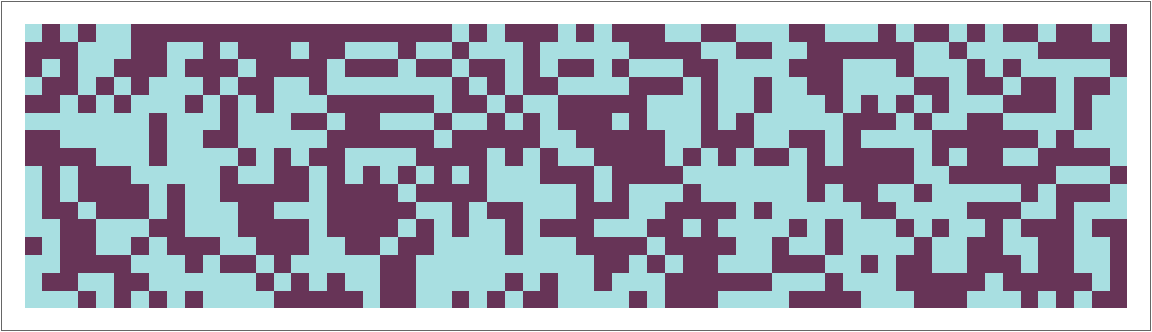Apply a chi square randomness test:

 In:=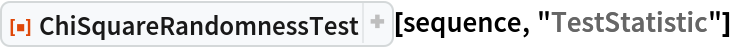Out=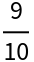In:=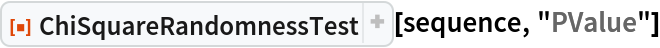Out=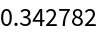Generate a sequence of random reals and test it for equidistribution:

 In:=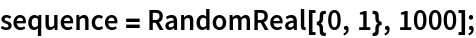Visualize the sequence:

 In:=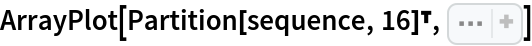Out=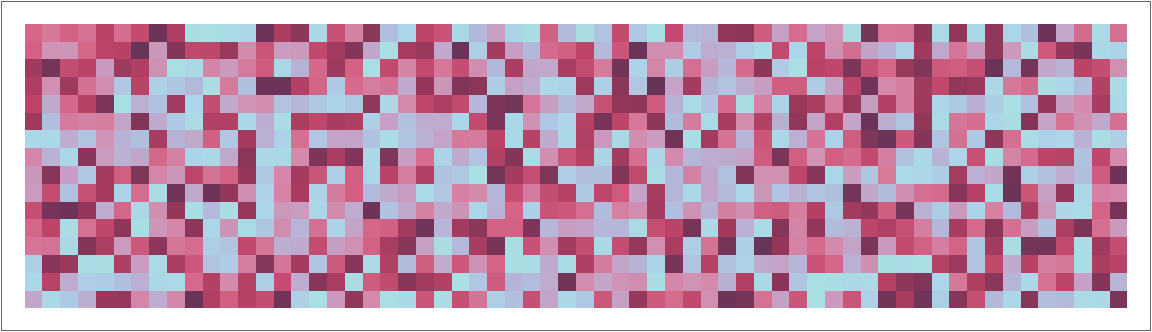Apply a chi square randomness test:

 In:=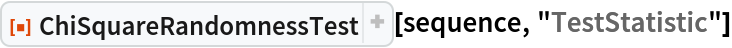Out=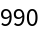In:=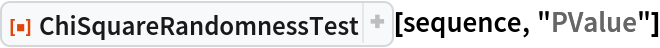Out=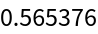Generate variates of the normal distribution and test if they are equidistributed:

 In:=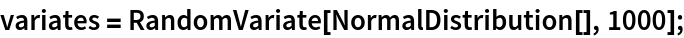Visualize the variates:

 In:=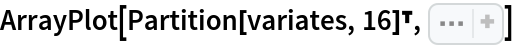Out=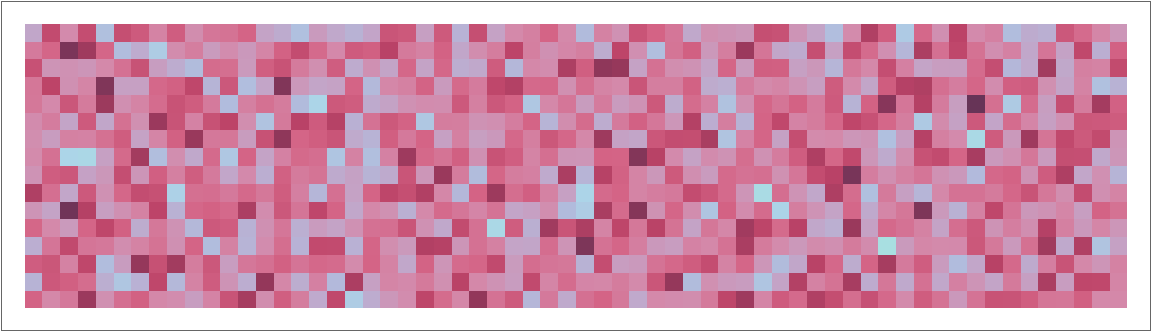In:=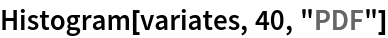Out=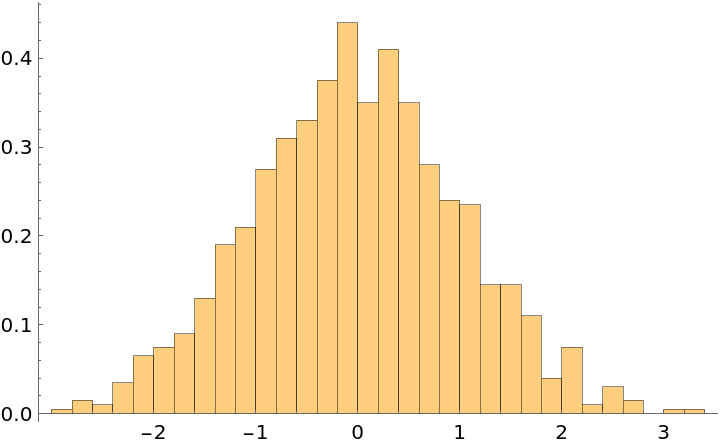Transform the variates so that the range is between 0 and 1:

 In:=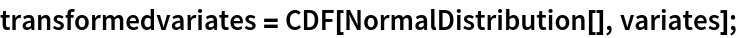Visualize the transformed variates:

 In:=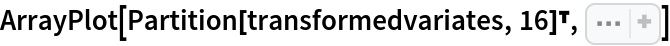Out=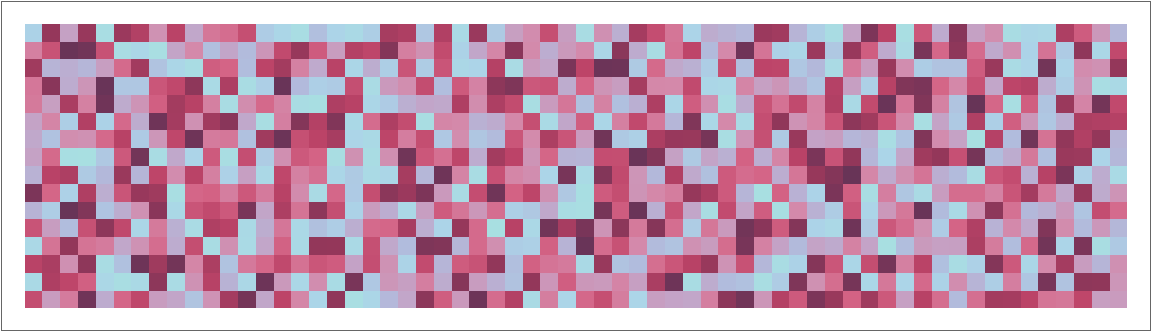In:=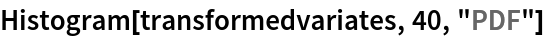Out=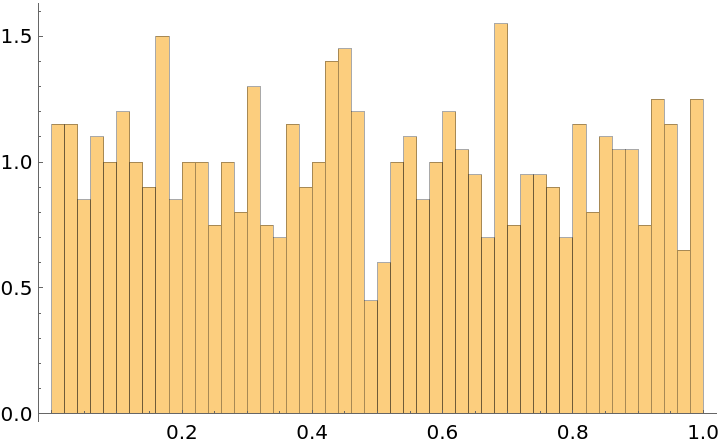Test if the transformed variates are equidistributed:

 In:=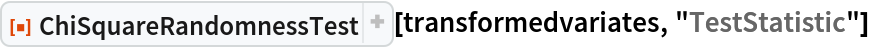Out=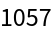In:=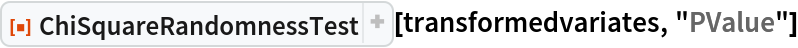Out=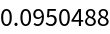### Applications (1)

Test to see if rule 30 is equidistributed:

 In:=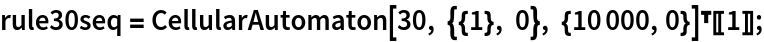In:=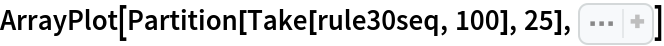Out=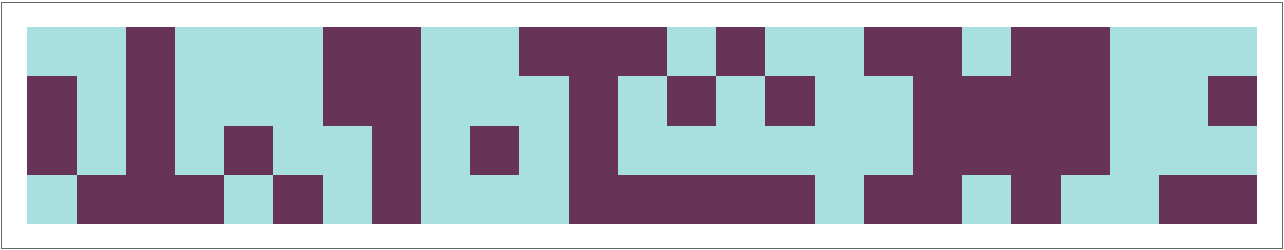In:=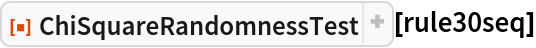Out=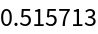Emmy Blumenthal

## Version History

• 1.0.0 – 08 July 2019

## Author Notes

The chi square test is meant to work with sequences of a certain length (typically longer than 30).